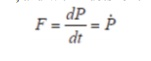Home | | Gas Dynamics and Jet Propulsion | Rocket Propulsion Thrust

# Rocket Propulsion Thrust

The basic principles of a rocket motor are relatively straightforward to understand. In rocketry the motor exists to accelerate the rocket, and thus it has to develop a force called “thrust”.

Thrust

The basic principles of a rocket motor are relatively straightforward to understand. In rocketry the motor exists to accelerate the rocket, and thus it has to develop a force called “thrust”. One of several definitions of force is that: Force = rate of change of momentum If we ignore (for a few paragraphs) any external effects we can say that the thrust is entirely due to the momentum of the propellant, a force called the “momentum thrust”. If we denote the thrust as F and the momentum as P, then mathematically:Sometimes for mathematical clarity we us the notation of P with a dot on top to denote the first derivative of P, and with 2 dots for the second derivative. Thus, in this new notation:You may also recall from the section on the rocket equation that momentum is the product of the mass and velocity. Thus we can say that the momentum of the flow from the nozzle of the rocket has a momentum:If the exhaust velocity remains constant, which is a reasonable assumption, we arrive at the equation:The term “m-dot” is known as the mass flow rate, in other words the rate at which mass is ejected through the nozzle in kg/sec. In other words this is the rate at which the rocket burns fuel. This is an interesting relationship, which can be expressed in words as: Momentum

Thrust = mass flow rate x exhaust velocity

Study Material, Lecturing Notes, Assignment, Reference, Wiki description explanation, brief detail
Mechanical : Gas Dynamics and Jet Propulsion : Space Propulsion : Rocket Propulsion Thrust |